Previous Article in Journal
TFR: A Novel Approach for Clock Synchronization Fault Recovery in Precision Time Protocol (PTP) Networks
Open AccessArticle

# Stability Augmentation of a Grid-Connected Wind Farm by Fuzzy-Logic-Controlled DFIG-Based Wind Turbines

1
Department of Electrical and Electronic Engineering, Kitami Institute of Technology (KIT), 165 Koen-cho, Kitami, Hokkaido 090-8507, Japan
2
Department of Electrical and Electronic Engineering, American International University-Bangladesh (AIUB), Ka-66/1, Kuratoli Road, Kuril, Khilkhet, Dhaka 1229, Bangladesh
3
Department of Electrical and Computer Engineering, Curtin University, Perth, WA 6845, Australia
*
Author to whom correspondence should be addressed.
Appl. Sci. 2018, 8(1), 20; https://doi.org/10.3390/app8010020
Received: 30 November 2017 / Revised: 17 December 2017 / Accepted: 19 December 2017 / Published: 24 December 2017

## Abstract

Wind farm (WF) grid codes require wind generators to have low voltage ride through (LVRT) capability, which means that normal power production should be resumed quickly once the nominal grid voltage has been recovered. However, WFs with fixed-speed wind turbines with squirrel cage induction generators (FSWT-SCIGs) have failed to fulfill the LVRT requirement, which has a significant impact on power system stability. On the other hand, variable-speed wind turbines with doubly fed induction generators (VSWT-DFIGs) have sufficient LVRT augmentation capability and can control the active and reactive power delivered to the grid. However, the DFIG is more expensive than the SCIG due to its AC/DC/AC converter. Therefore, the combined use of SCIGs and DFIGs in a WF could be an effective solution. The design of the rotor-side converter (RSC) controller is crucial because the RSC controller contributes to the system stability. The cascaded control strategy based on four conventional PI controllers is widely used to control the RSC of the DFIG, which can inject only a small amount of reactive power during fault conditions. Therefore, the conventional strategy can stabilize the lower rating of the SCIG. In the present paper, a new control strategy based on fuzzy logic is proposed in the RSC controller of the DFIG in order to enhance the LVRT capability of the SCIG in a WF. The proposed fuzzy logic controller (FLC) is used to control the reactive power delivered to the grid during fault conditions. Moreover, reactive power injection can be increased in the proposed control strategy. Extensive simulations executed in the PSCAD/EMTDC environment for both the proposed and conventional PI controllers of the RSC of the DFIG reveal that the proposed control strategy can stabilize the higher rating of the SCIG.

## 1. Introduction

Emerging environmental concerns and attempts to curtail the dependence on fossil fuel resources are bringing renewable energy resources into the mainstream of the electric power sector. Among the various renewable resources, wind power is the most promising from both technical and economic standpoints. The new global total for wind power at the end of 2015 was 432.9 GW, which represents a cumulative market growth of more than 17% . By 2030, wind power could reach 2110 GW and supply up to 20% of the global electricity . This large penetration of wind power into the existing grid has introduced some vulnerabilities to the power grid. In order to maintain the stability of the power system and ensure smooth operation, low voltage ride through (LVRT) requirements have been imposed around the world . In the event of a fault, LVRT mandates that wind farms (WFs) stay connected to the grid in order to support the grid in the same manner as conventional synchronous generators (SGs).
Most wind turbines are constructed using fixed-speed wind turbines with squirrel cage induction generators (FSWT-SCIGs). SCIGs have some advantageous characteristics, such as simplicity, robust construction, low cost, and operational simplicity . However, FSWT-SCIGs are connected directly to the grid and have no LVRT capabilities during voltage dips . Moreover, the FSWT-SCIG requires a large reactive power in order to recover air gap flux when a short circuit fault occurs in the power system. If sufficient reactive power is not supplied, the electromagnetic torque of the SCIG decreases significantly. As a result, the rotor speed of the SCIG increases significantly and can make the power system unstable . Reactive power compensation is a major issue, especially for FSWT-SCIGs. A capacitor bank is usually used to meet the reactive power compensation requirement of an SCIG. However, the SCIG requires more reactive power during fault conditions than in the steady state, and the capacitor bank is not able to supply more reactive power during transient conditions.
A static synchronous compensator (STATCOM) , superconducting magnetic energy storage (SMES) , and an energy capacitor system (ECS) , for example, are installed in WFs with FSWT-SCIGs in order to improve the LVRT capability during a fault condition. However, the overall system cost increases.
On the other hand, variable-speed wind turbines with doubly fed induction generators (VSWT-DFIGs) have some advantageous characteristics, such as light weight, higher output power and efficiency, lower cost, variable-speed operation, and smaller size. In addition to the lower power electronic converter rating required by the DFIG, compared to permanent magnet synchronous generators (PMSGs) , the recent price upsurge of permanent magnet materials has given the DFIG another advantage over the PMSG . In addition, the DFIG has better system stability characteristics than the SCIG during fault conditions, because of its capability for independent control of active and reactive power delivered to the grid . By taking advantage of DFIG reactive power control, it is possible to stabilize the SCIG in a WF. Thus, reactive power compensation can be implemented at lower cost. The partial converter is connected to the rotor terminal of the DFIG via slip rings. The converter consists of a rotor-side converter (RSC) and a grid-side converter (GSC). As reported in previous studies [10,11,12], various control strategies can be adopted for both the RSC and the GSC. However, the design procedure of the RSC is very crucial because it is controlling active and reactive power delivered to the grid.
Some auxiliary hardware circuits have been used to help the DFIG to improve the LVRT requirement. For example, the rotor crowbar circuit is used in the rotor terminals to isolate the RSC from the rotor circuit [13,14]. However, the rotor crowbar circuit converts the DFIG to a simple induction machine, which absorbs reactive power from the grid. A chopper circuit and parallel capacitors are used to smooth the DC-link voltage by dissipating the excessive power in the DC-link circuit [15,16]. Dynamic braking resistors connected to the stator  and a bridge type fault current limiter  are used to limit the stator and rotor overcurrents. A series-connected converter  and a dynamic voltage restorer  are used to keep the stator voltage constant under grid faults. In previous studies [21,22], static VAR compensators or STATCOMs were used to supply extra reactive power to the grid during grid faults. Although the LVRT capability is enhanced through various types of equipment [13,14,15,16,17,18,19,20,21,22], this equipment requires additional converters or equipment, which increases the complexity and cost of the wind turbine system and decreases its reliability.
The cascaded control system for the RSC described in  is also used to improve LVRT capability, where several PI controllers are used in the inner and outer loops. However, due to changes in the parameters of the grid during fault conditions, the conventional PI controller with a fixed gain is not sufficient to ensure the system stability of a large power system. The setting of the parameters of the PI controllers used in cascaded control is cumbersome, especially in power system applications that are difficult to express as a mathematical model or a transfer function. In , a Taguchi approach for optimum design of PI controllers in a cascaded control scheme was presented. However, this cascaded control strategy with the conventional PI controller in the inner loop cannot provide a large amount of reactive power. Thus, the strategy can stabilize only lower ratings of the SCIG. Therefore, using an fuzzy logic controller (FLC) in the inner loop of the rotor-side controller to more efficiently provide reactive power during fault periods is convenient. The FLC can handle nonlinear systems very effectively because it offers variable gain during transient conditions. Thus, the DFIG controlled by the FLC can stabilize a larger amount of SCIG. Moreover, the overall system cost can be decreased by incorporating a lower rating of the DFIG along with a higher rating of the SCIG. This is one of the novel features of the present paper.
Therefore, the main contribution of the present paper is the design of a new control strategy based on fuzzy logic in the inner loop of the rotor-side controller for the DFIG to improve the LVRT capability and increase the capacity of the SCIG-based WF. Detailed modeling and control strategies of the overall system are presented. In order to evaluate the effectiveness of the proposed controller, transient and dynamic analyses are performed. Real wind-speed data measured on Rishiri Island, Hokkaido, Japan are considered in the dynamic analysis.
The transient performance of the overall system composed of SGs, an FLC-controlled DFIG, and an SCIG is compared with that composed of a DFIG with the conventional PI-controlled RSC presented in . Finally, the proposed control strategy is found to be very effective for ensuring the stability of a large power system. Moreover, the capacity of the installed SCIG can be increased.
The remainder of the present paper is organized as follows. Section 2 presents the wind turbine model. Section 3 presents the DFIG model, and the design procedure of the proposed FLC is introduced in Section 4. Section 5 deals with the power system model. Section 6 briefly describes the LVRT requirements for wind power. The simulation results and a discussion of the performance of the proposed and conventional methods are presented in Section 7. Finally, Section 8 summarizes the findings and concludes the paper.

## 2. Wind Turbine Model

In the wind turbine model, the aerodynamic power output is given as follows :
$P w = 0.5 ρ π R 2 V w 3 C p ( λ , β ) ,$
where Pw is the captured wind power, ρ is the air density (KG/m3), R is the radius of the rotor blade (m), Vw is the wind speed (m/s), and Cp is the power coefficient.
The value of Cp can be calculated as follows :
$C p ( λ , β ) = c 1 ( c 2 λ i − c 3 β − c 4 ) e − c 5 λ i + c 6 λ$
$1 λ i = 1 λ − 0.08 β − 0.035 β 3 + 1$
$λ = ω r R V w$
$T w = P w ω r ,$
where Tw is the wind turbine torque, β is the pitch angle, and λ is the tip speed ratio. Moreover, c1 through c6 are the characteristic coefficients of the wind turbine (c1 = 0.5176, c2 = 116, c3 = 0.4, c4 = 5, c5 = 21, and c6 = 0.0068) , and ωr is the rotational speed of the wind turbine (rad/s).
The Cp vs λ characteristics shown in Figure 1 are obtained using Equation (2) with different values of the pitch angle (β). When β is equal to zero degrees, the optimum power coefficient (Cpopt) is 0.48, and the optimum tip speed ratio (λopt) is 8.1.
Figure 2 and Figure 3 show the models of the blade pitch control system for FSWT and VSWT , respectively. In FSWT, the pitch control system is used to control the power output of the SCIG so as not to exceed the rated power. In VSWT, the rotor speed of DFIG is regulate by the pitch controller so as not to exceed the rated speed. The control loop of the pitch actuator is represented by a first-order transfer function with a pitch rate limiter. A PI controller is used to manage the tracking error.
Figure 4 shows the maximum power point tracking (MPPT) curve for the VSWT-DFIG.

## 3. DFIG Model

The configuration of the VSWT-DFIG system, along with its control system, is shown in Figure 5. The model consists of a wind turbine model with aerodynamic characteristics, a pitch controller, a wound rotor induction generator (WRIG), and an AC/DC/AC converter based on two levels of insulated gate bipolar transistors (IGBTs), which are controlled by the rotor-side controller and the grid-side controller, respectively.
The wind turbine drives the WRIG to convert wind power into electrical power. The rotational speed (ωr) is obtained from the rotor of the WRIG. A pitch controller is used to control the blade pitch angle of the wind turbine in order to reduce the output power when the rotational speed exceeds the rated speed. The WRIG model available in the PSCAD library is used in the present study . The rotor position (θr) is derived from the rotor of the WRIG. As indicated by the configuration of the VSWT-DFIG system, the stator terminal is directly connected to the grid system. The AC/DC/AC converter is installed between the rotor of the WRIG and the grid system. The rating of the converter is 30% of the WRIG rating. The pulse width modulation (PWM) technique is used to generate the necessary gate pulses for driving the AC/DC/AC converter. The carrier frequency is taken as 3.0 kHz. The RSC is connected to the rotor winding of the WRIG, which provides variable frequency excitation depending on the wind-speed condition. The GSC is connected to the grid system through a transformer. A protection system with a DC chopper is installed in the DC-link circuit. The DC chopper is controlled by the comparator block, which triggers the DC chopper switch when the DC-link voltage becomes greater than or equal to the predefined limit (Vdc ≥ 1.15 pu).

#### 3.1. Conventional Rotor-Side Controller

The conventional cascaded controller for the RSC is presented in . This controller consists of four conventional PI controllers to compensate different error signals. The reference reactive power (Qdfig*) is set to zero for unity power factor operation. The active power and reactive power delivered to the grid are controlled using q-axis and d-axis rotor currents, respectively.

#### 3.2. Proposed Rotor-Side Controller

The proposed controller for the RSC is depicted in Figure 6. This controller consists of three PI controllers and one FLC. The main motivation behind using one FLC in the inner loop of the cascaded controller is maximization of the reactive power injection. The FLC offers variable gain depending on the system parameters. Due to the variable gain, the FLC can inject reactive power (Qdfig) more effectively in the fault condition. Thus, the grid voltage can quickly be retraced back to the nominal value. Moreover, the FLC can stabilize a higher rating of the SCIG as compared to the conventional PI-based controller of the RSC in the inner loop.
The active power (Pdfig) and reactive power (Qdfig) outputs of the DFIG are controlled by regulating the rotor winding current. The reference active power (Pref) is calculated by subtracting the losses (Ploss) from the MPPT output (Pmppt). In the upper loop portion, the grid voltage (Vg) is taken as feedback to regulate the terminal voltage constant at 1.0 pu. The q-axis current (Irq) controls the active power delivered to the grid, and the d-axis current (Ird) controls the reactive power delivered to the grid.
In the normal operating condition (Vg > 0.9 pu), the RSC regulates the active power delivered to the grid. During a fault condition (Vg < 0.9 pu), a comparator sends a signal so that active power transfer to the grid becomes zero. By controlling the power in this manner, the reactive power injected to the grid can be maximized.
The detailed design procedure of the FLC will be discussed in Section 4.

#### 3.3. Grid-Side Controller

The controller for the GSC is depicted in Figure 7. This controller consists of four PI controllers to compensate different error signals. The GSC reactive power (Qg) and DC-link voltage (Vdc) are controlled through d-axis (Igd) and q-axis (Igq) current components, respectively. The reactive power reference is set to zero, and the DC-link voltage reference is set to 1.0 pu (1.2 kV).

## 4. Fuzzy Logic Controller Design

Figure 8 shows a block diagram of the proposed FLC. The FLC is composed of fuzzification, a membership function, a rule base, a fuzzy inference, and defuzzification, as shown in Figure 9.
In order to design the proposed FLC, the error of the rotor d-axis current (eIrd) and rate of change of the eIrd (d[eIrd]/dt) are considered as the controller inputs. The reference rotor d-axis voltage (Vrd*) is chosen as the controller output. In Figure 8, 1/z is one sampling time delay.
The triangular membership functions with overlap used for the input and output fuzzy sets are shown in Figure 10, where linguistic variables are indicated as NB (Negative Big), NM (Negative Medium), NS (Negative Small), ZO (Zero), PS (Positive Small), PM (Positive Medium), and PB (Positive Big).
The rules of fuzzy mapping of the input variables to the output are given in the following form:
IF <eIrd is PB> and <d(eIrd)/dt is NS> THEN <Vrd* is PS>
IF <eIrd is NM> and <d(eIrd)/dt is NS> THEN <Vrd* is NB>
The entire rule base is listed in Table 1, which includes a total of 49 rules.
In the present study, Mamdani’s max-min method is used as the inference mechanism . The center of gravity method is used for defuzzification in order to obtain Vrd* .

## 5. Power System Model

The power system model used for transient stability analysis is shown in Figure 11. The model is composed of a nine-bus main system  and a WF. The main system is composed of three conventional power plants: two thermal power plants (SG1 and SG2) and one hydropower plant (SG3). Both SG1 and SG3 are operated under automatic generation control (AGC), and SG2 is operated under governor-free (GF) control. The parameters of the SGs are listed in Table 2. The IEEE type AC4A excitation system model shown in Figure 12 is considered for all SGs . Table 3 lists the parameters taken from . Figure 13 shows a block diagram of the reheat steam turbine governor system used in the thermal power plants (SG1 and SG2) . The hydro turbine governor model system used for the hydropower plant (SG3) is shown in Figure 14 . The parameters of both turbine systems are presented in Table 4 . For AGC operation, an integral controller is installed on the governor system for both SG1 and SG3.
The integral controller on selected units for AGC is shown in Figure 15 . The output of the AGC supplies the power load reference of the governor system depending on the speed deviation of the SG (Δωsg). The integral gain Ki is set to 6.
A WF is connected to the main system at bus 5, as shown in Figure 11, and consists of one VSWT-DFIG and one FSWT-SCIG. In order to reduce computational time, each wind generator is represented as an aggregated equivalent single machine [31,32]. The total capacity of the WF is 100 MW. A capacitor bank (C) is used for reactive power compensation of the SCIG. The value of C is chosen such that the power factor of the SCIG-based wind generator becomes unity at the rated operating condition. The base power of the system is 100 MVA, and the rated frequency is 50 Hz. The parameters of the DFIG and the SCIG are presented in Table 5.

## 6. LVRT Requirement for Wind Power

The requirement of LVRT for wind power is depicted in Figure 16 . The WF must remain connected to the grid if the voltage drop is within the defined r.m.s. value and its duration is also within the defined period, as shown in the figure. If the voltage of the connection point recovers to 90% of the rated voltage within 1.5 s following the voltage drop, all wind turbines within the WF shall stay online without tripping.

## 7. Simulation Results and Discussions

#### 7.1. Transient Stability Analysis

Simulation analysis is performed on the model system shown in Figure 11 using PSCAD/EMTDC software. The FORTRAN language is incorporated into PSCAD/EMTDC in order to implement FLC as new component. The simulation time is chosen as 10 s. The triple-line-to-ground (3LG) fault near bus 11 is considered to be a network disturbance, as shown in Figure 11. The fault occurs at 0.1 s. The duration of the fault is 0.1 s. The circuit breakers (CBs) on the faulted line are opened at 0.2 s in order to isolate the faulty line from the power system. The CBs are reclosed at 1.0 s based on the consideration that the fault has been cleared. The wind speed data applied to each wind turbine is maintained constant at the rated speed based on the assumption that the wind speed does not change dramatically within this small period of time. Simulation analyses are carried out for both the proposed and conventional rotor-side controllers reported in  in order to demonstrate the effectiveness of the proposed control system. The simulation results are presented and discussed in the following subsections.

#### 7.1.1. Analysis Using the Conventional Rotor-Side Controller

Two cases are considered using the conventional rotor-side controller. The parameters for conventional PI controllers are chosen based on the method presented in the literature . The power rating of each wind generator in Case 01 is DFIG = 59 MW and SCIG = 41 MW (total: 100 MW), and, in Case 02, DFIG = 58 MW and SCIG = 42 MW (total: 100 MW). Different power ratings of the wind generators are chosen, because the objective is to stabilize the maximum possible rating of SCIG by using lowest possible rating of DFIG, while the total capacity of WF is kept constant at 100 MW. In this present study, it is calculated by running the simulation for multiple times with different combinations of power ratings of the wind generators.
Figure 17a,b show the responses of reactive powers, which indicates that the DFIG can provide the necessary reactive power during the severe symmetrical 3LG fault in Case 01. As a result, the connection point voltage recovers to the rated value quickly in Case 01, as shown in Figure 18a. However, in Case 02, the DFIG does not provide the necessary reactive power during the fault condition. Thus, the connection point voltage cannot recover to the rated value. Since the connection point voltage does not satisfy the standard grid code of Figure 16 in Case 02, the WF is disconnected from the power system by opening CBs near bus 12 at 2 s. The rotor speed responses of both wind generators are stable in Case 01, but unstable in Case 02, as shown in Figure 19.
Figure 20 shows the active power output of DFIG and SCIG, respectively. The active power can recover to the nominal value in Case 01 for both wind generators, but failed to recover to the nominal value in Case 02. Moreover, the DC-link voltage of the DFIG becomes more stable in Case 01, as compared to Case 02, as shown in Figure 21a.
Figure 22a,b show the active power output and rotor speed responses, respectively, of the conventional power plants (SGs). The active power and rotational speed of the SGs can return to the initial condition in Case 01. However, the active power of the SGs in Case 02 increases significantly after the WF has been disconnected, resulting in a rotor speed drop of the SGs. It is clear that the system becomes unstable in Case 02, which can also be seen from Figure 23, where the system frequency collapses in Case 02 after the WF has been disconnected.
Therefore, the lowest power rating of the DFIG with the conventional rotor-side controller is 59 MW in order to stabilize the 41 MW SCIG. The DFIG can also stabilize the SGs.

#### 7.1.2. Analysis Using the Proposed Rotor-Side Controller

Two cases are considered using the proposed rotor-side controller shown in Figure 6. The power rating of each wind generator in Case 01 is DFIG = 28 MW and SCIG = 72 MW (total: 100 MW), and, in Case 02, DFIG = 27 MW and SCIG = 73 MW (total: 100 MW).
Figure 24a,b show the responses of reactive powers, which indicate that the DFIG can provide the necessary reactive power during the severe symmetrical 3LG fault in Case 01. As a result, the connection point voltage quickly recovers to the rated value in Case 01, as shown in Figure 25a. However, in Case 02, the DFIG does not provide the necessary reactive power during the fault condition, and thus, the connection point voltage cannot be back to the rated value. Since the connection point voltage does not satisfy the standard grid code of Figure 16 in Case 02, the WF is disconnected from the power system by opening CBs near bus 12 at 2 s. The rotor speed responses of both wind generators are stable in Case 01, but unstable in Case 02, as shown in Figure 26.
Figure 27 shows the active power output of DFIG and SCIG, respectively. The active power can recover to the nominal value in Case 01 for both wind generators, but failed to recover to the nominal value in Case 02. Moreover, the DC-link voltage of the DFIG becomes more stable in Case 01, as compared to Case 02, as shown in Figure 28a. Figure 29a,b show the active power output and rotor speed responses, respectively, of the conventional power plants (SGs). The active power and rotational speed of the SGs can return to the initial condition in Case 01. However, the active power of the SGs in Case 02 increases significantly after the WF has been disconnected, resulting in a rotor speed drop of the SGs. It is clear that the system becomes unstable in Case 02, which can also be seen from Figure 30, where the system frequency collapses in Case 02 after the WF has been disconnected.
Therefore, the lowest power rating of the DFIG with the proposed rotor-side controller is 28 MW in order to stabilize the 72 MW SCIG. The DFIG can also stabilize the SGs. Finally, the reactive power output of DFIG (capacity is 28 MW) for both proposed and conventional rotor-side controllers is depicted in Figure 31. The reactive power output for the proposed FLC-controlled DFIG is larger and more efficient than the conventional PI-controlled DFIG.

#### 7.2. Dynamic Performance Analysis Using the Proposed Rotor-Side Controller

In order to evaluate the dynamic performance of the proposed system, the real wind speed data measured at Rishiri Island, Hokkaido, Japan, shown in Figure 32, is used in the simulation. The power system model shown in Figure 11 is considered in this dynamic analysis. The capacities of the DFIG and the SCIG are 28 MW and 72 MW (The total capacity of the WF is 100 MW), respectively. Because this power ratings of the wind generators are stable case for the proposed system as presented in Section 7.1.2.
Figure 33 shows the reactive power output of wind generators. The DFIG provides the necessary reactive power to the SCIG for voltage regulation. Thus, the connection point voltage at bus 12 is approximately constant, as shown in Figure 34. Figure 35 shows the active power outputs of the VSWT-DFIG and the FSWT-SCIG. The DC-link voltage of the DFIG is maintained constant, as shown in Figure 36. The variation of the DC-link voltage is very small, even though there are wide fluctuations in the wind speed. Figure 37 shows the responses of the blade pitch angle. The increase in the blade pitch angle will help to reduce the mechanical power extraction from the wind turbines. The total active and reactive power output of the wind generators at bus 12 is shown in Figure 38.
The active power output of conventional power plants (SGs) and the power system frequency response are shown in Figure 39 and Figure 40. The conventional SGs adjust their active power output according to the fluctuating power injected from the WF. Moreover, the frequency variation lies within the permissible limit in Japan (±0.2 Hz).

#### 7.3. Discussion

The transient simulation analyses in Section 7.1.1 and Section 7.1.2 reveal that the necessary power rating of the DFIG to stabilize the SCIG in the WF, as well as to prevent conventional SGs from becoming out of step during a 3LG fault, is much lower in the case of the proposed rotor-side controller than in the case of the conventional rotor-side controller, where the total capacity of the DFIG and the SCIG is 100 MW. Table 6 summarizes the results, which reveal that, for stable operation of the WF and SGs, the lowest power rating of the DFIG is 28 MW for the proposed method and 59 MW for the conventional method.
The dynamic simulation analysis confirmed that the proposed FLC-controlled DFIG can effectively inject reactive power and thus maintain the terminal voltage constant under a randomly varying wind speed.

## 8. Conclusions

In order to enhance the LVRT performance of the SCIG-based WF, partial installation of the DFIG with the new rotor-side controller based on the FLC is proposed in the present study. Moreover, a comparative study of the proposed and conventional rotor-side controllers is carried out. Based on the simulation results and performance analyses, the following points are of notable significance regarding the proposed method:
• The proposed FLC-controlled DFIG of a lower power rating can stabilize the larger power rating of SCIG as well as conventional SGs during fault conditions.
• The installation cost can be decreased by incorporating a small number of VSWT-DFIGs with the proposed controller and a large number of FSWT-SCIGs into a WF.
• The proposed FLC controlled DFIG system can maintain its terminal voltage at constant under normal operating conditions by effectively injecting reactive power into the grid.
Therefore, if the proposed DFIG with a relatively small power rating is installed at a WF composed mainly of SCIGs, its LVRT capability, as well as the stability of a connected power system, can be enhanced.

## Acknowledgments

The present study was supported by a Grant-in-Aid for Scientific Research (B) from the Ministry of Education, Science, Sports, and Culture of Japan.

## Author Contributions

Md. Rifat Hazari and Junji Tamura developed the theoretical concepts, designed the power system model and proposed RSC controller, and performed the simulation analysis. Md. Rifat Hazari and S. M. Muyeen developed the fuzzy logic controller. Mohammad Abdul Mannan, S. M. Muyeen, Atsushi Umemura, Rion Takahashi, and Junji Tamura revised the manuscript. Md. Rifat Hazari wrote the manuscript.

## Conflicts of Interest

The authors declare no conflict of interest.

## Abbreviations

 FSWT fixed-speed wind turbine SCIG squirrel cage induction generators WF wind farm LVRT low-voltage ride through VSWT variable-speed wind turbine DFIG doubly fed induction generators RSC rotor-side converter GSC grid-side converter FLC fuzzy logic controller SG synchronous generator STATCOM static synchronous compensator SMES superconducting magnetic energy storage ECS energy capacitor system PMSG permanent magnet synchronous generator MPPT maximum power point tracking WRIG wound rotor induction generator IGBT insulated gate bipolar transistor PWM pulse width modulation NB negative big NM negative medium NS negative small ZO zero PS positive small PM positive medium PB positive big AGC automatic generation control GF governor free 3LG triple-line-to-ground fault CB circuit breaker Pw captured wind power ρ air density (KG/m3) R radius of the rotor blade (m) Vw wind speed (m/s) Cp power coefficient Tw wind turbine torque β pitch angle λ tip speed ratio Cpopt optimum power coefficient λopt optimum tip speed ratio ωr rotational speed θr rotor position Vdc DC-link voltage Vdc* reference DC-link voltage Cdc DC-link capacitor Pdfig active power output of DFIG Qdfig reactive power output of DFIG Pref reference active power Qdfig* reference reactive power Ploss power losses Pmppt MPPT output Vg grid voltage Vg* grid voltage reference Isa, Isb, Isc stator currents for phases A, B, and C Isd, Isq stator d-axis and q-axis currents Ira, Irb, Irc rotor currents for phases A, B, and C Ird, Irq rotor d and q axis currents Iga, Igb, Igc grid currents for phases A, B, and C Igd, Igq grid d-axis and q-axis currents eIrd error of rotor d-axis current d(eIrd)/dt change in the error of the rotor d-axis current 1/z one sampling time delay

## References

1. Global Wind Energy Council (GWEC). Annual Market Update 2015, Global Wind Report. 2015. Available online: http://www.gwec.net/ (accessed on 15 October 2017).
2. Global Wind Energy Council (GWEC). Global Wind Energy Outlook 2016: Wind Power to Dominate Power Sector Growth. 2016. Available online: http://www.gwec.net/ (accessed on 15 October 2017).
3. Tsili, M.; Papathanassiou, S. A Review of Grid Code Technical Requirements for Wind Farms. IET Renew. Power Gener. 2009, 3, 308–332. [Google Scholar] [CrossRef]
4. Muyeen, S.M.; Tamura, J.; Murata, T. Stability Augmentation of a Grid Connected Wind Farm; Springer: London, UK, 2009. [Google Scholar]
5. Suul, J.A.; Molinas, M.; Undeland, T. STATCOM-based Indirect Torque Control of Induction Machines during Voltage Recovery after Grid Faults. IEEE Trans. Power Electron. 2010, 25, 1240–1250. [Google Scholar] [CrossRef]
6. Yu, J.; Duan, X.; Tang, Y.; Yuan, P. Control Scheme Studies of Voltage Source Type Superconducting Magnetic Energy Storage (SMES) Under Asymmetrical Voltage. IEEE Trans. Appl. Supercond. 2002, 12, 750–753. [Google Scholar]
7. Muyeen, S.M.; Takahashi, R.; Ali, M.H.; Murata, T.; Tamura, J. Transient Stability Augmentation of Power Systems including Wind Farms using ECS. IEEE Trans. Power Syst. 2008, 23, 1179–1187. [Google Scholar] [CrossRef]
8. Tripathi, S.; Tiwari, A.; Singh, D. Grid-integrated Permanent Magnet Synchronous Generator based Wind Energy Conversion Systems: A Technology Review. Renew. Sustain. Energy Rev. 2015, 51, 1288–1305. [Google Scholar] [CrossRef]
9. Boldea, I.; Tutelea, L.; Blaabjerg, F. High Power Wind Generator Designs with Less or No PMs: An Overview. In Proceedings of the International Conference on Electrical Machines and Systems (ICEMS), Hangzhou, China, 22–25 October 2014; pp. 1–14. [Google Scholar]
10. Rosyadi, M.; Umemura, A.; Takahashi, R.; Tamura, J.; Uchiyama, N.; Ide, K. Simplified Model of Variable Speed Wind Turbine Generator for Dynamic Simulation Analysis. IEEJ Trans. Power Energy 2015, 135, 538–549. [Google Scholar] [CrossRef]
11. Okedu, K.; Muyeen, S.M.; Takahashi, R.; Tamura, J. Wind Farm Stabilization by using DFIG with Current Controlled Voltage Source Converter taking Grid Codes into Consideration. IEEJ Trans. Power Energy 2012, 132, 251–259. [Google Scholar] [CrossRef]
12. Bourdoulis, M.K.; Alexandridis, A.T. Direct Power Control of DFIG Wind Systems Based on Nonlinear Modeling and Analysis. IEEE J. Emerg. Sel. Top. Power Electron. 2014, 2, 764–775. [Google Scholar] [CrossRef]
13. Pannell, G.; Atkinson, D.J.; Zahawi, B. Minimum-threshold Crowbar for a Fault-ride-through Grid-code-Compliant DFIG Wind Turbine. IEEE Trans. Energy Convers. 2010, 25, 750–759. [Google Scholar] [CrossRef]
14. Vidal, J.; Abad, G.; Arza, J.; Aurtenechea, S. Single Phase DC Crowbar Topologies for Low Voltage Ride Through Fulfillment of High-power Doubly Fed Induction Generator-based Wind Turbines. IEEE Trans. Energy Convers. 2013, 28, 768–781. [Google Scholar] [CrossRef]
15. Pannell, G.; Zahawi, B.; Atkinson, D.J.; Missailidis, P. Evaluation of the Performance of a DC-link Brake Chopper as a DFIG Low-Voltage Fault-Ride-Through Device. IEEE Trans. Energy Convers. 2013, 28, 535–542. [Google Scholar] [CrossRef]
16. Huchel, L.; Moursi, M.S.E.; Zeineldin, H.H. A Parallel Capacitor Control Strategy for Enhanced FRT Capability of DFIG. IEEE Trans. Sustain. Energy 2015, 6, 303–312. [Google Scholar] [CrossRef]
17. Okedu, K.E.; Muyeen, S.M.; Takahashi, R.; Junji, T. Wind Farms Fault Ride Through using DFIG with New Protection Scheme. IEEE Trans. Sustain. Energy 2012, 3, 242–254. [Google Scholar] [CrossRef]
18. Guo, W.; Xiao, L.; Dai, S.; Xu, X.; Li, Y.; Wang, Y. Evaluation of The Performance of BTFCLs for Enhancing LVRT Capability of DFIG. IEEE Trans. Power Electron. 2015, 30, 3623–3637. [Google Scholar] [CrossRef]
19. Zhang, S.; Tseng, K.J.; Choi, S.S.; Nguyen, T.D.; Yao, D.L. Advanced Control of Series Voltage Compensation to Enhance Wind Turbine Ride Through. IEEE Trans. Power Electron. 2012, 27, 763–772. [Google Scholar] [CrossRef]
20. Ibrahim, A.O.; Nguyen, T.H.; Lee, D.C.; Kim, S.C. A Fault Ride through Technique of DFIG Wind Turbine Systems using Dynamic Voltage Restorers. IEEE Trans. Energy Convers. 2011, 26, 871–882. [Google Scholar] [CrossRef]
21. Amaris, H.; Alfonso, M. Coordinated Reactive Power Management in Power Networks with Wind Turbines and FACTS Devices. Energy Convers. Manag. 2011, 52, 2575–2586. [Google Scholar] [CrossRef]
22. Qiao, W.; Harley, R.G.; Venayagamoorthy, G.K. Coordinated Reactive Power Control of a Large Wind Farm and a STATCOM using Heuristic Dynamic Programming. IEEE Trans. Energy Convers. 2009, 24, 493–503. [Google Scholar] [CrossRef]
23. Hasanien, H.M.; Muyeen, S.M. A Taguchi Approach for Optimum Design of Proportional-Integral Controllers in Cascaded Control Scheme. IEEE Trans. Power Syst. 2013, 28, 1636–1644. [Google Scholar] [CrossRef]
24. Matlab Documentation Center. Available online: http://www.mathworks.co.jp/jp/help/ (accessed on 20 December 2016).
25. Liu, J.; Rosyadi, M.; Umemura, A.; Takahashi, R.; Tamura, J. A Control Method of Permanent Magnet Wind Generators in Grid Connected Wind Farm to Damp Load Frequency Oscillation. IEEJ Trans. Power Energy 2014, 134, 393–398. [Google Scholar] [CrossRef]
26. Manitoba HVDC Research Center. PSCAD/EMTDC User’s Manual; Manitoba HVDC Research Center: Winnipeg, MB, Canada, 1994; Available online: https://hvdc.ca/uploads/ck/files/reference _material/EMTDC_User_Guide_v4_2_1.pdf (accessed on 21 October 2017).
27. Mamdani, E.M. Applications of Fuzzy Algorithms for Simple Dynamic Plants. Proc. IEEE 1974, 21, 1585–1588. [Google Scholar] [CrossRef]
28. Driankov, D.; Hellendoorn, H.; Reinfrank, M. An Introduction to Fuzzy Control; Springer: Berlin, Germany, 1993. [Google Scholar]
29. Anderson, P.M.; Fouad, A.A. Power System Control & Stability; John Wiley & Sons: Oxford, UK, 2008. [Google Scholar]
30. Kundur, P. Power System Stability & Control; McGraw-Hill Inc.: New York, NY, USA, 1994. [Google Scholar]
31. Ackerman, T. Wind Power in Power System; John Wiley & Sons: Oxford, UK, 2005. [Google Scholar]
32. WECC Renewable Energy Modeling Task Force. WECC Wind Power Plant Dynamic Modeling Guide; WECC Renewable Energy Modeling Task Force, 2014. Available online: https://www.wecc.biz/Reliability/WECC%20Wind%20Plant%20Dynamic%20Modeling%20Guidelines.pdf (accessed on 21 October 2017).
33. E. ON NETZ GmbH. Grid Connection Regulation for High and Extra High Voltage; E. ON NETZ GmbH: Essen, Germany, 2006. [Google Scholar]
Figure 1. Cp vs λ characteristics of the wind turbine for various pitch angles.
Figure 1. Cp vs λ characteristics of the wind turbine for various pitch angles.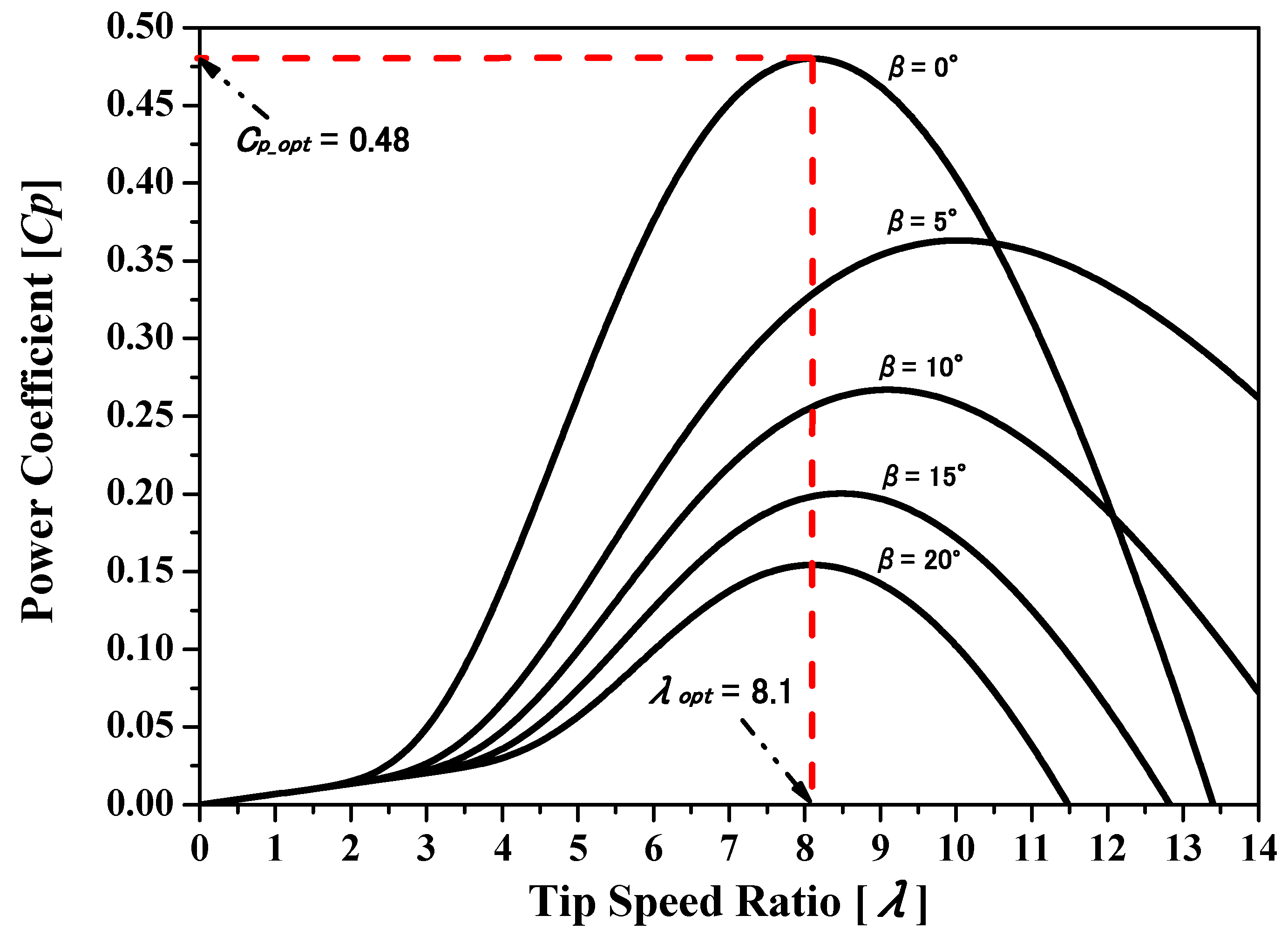Figure 2. Pitch controller for fixed-speed wind turbine (FSWT).
Figure 2. Pitch controller for fixed-speed wind turbine (FSWT).Figure 3. Pitch controller for variable-speed wind turbine (VSWT).
Figure 3. Pitch controller for variable-speed wind turbine (VSWT).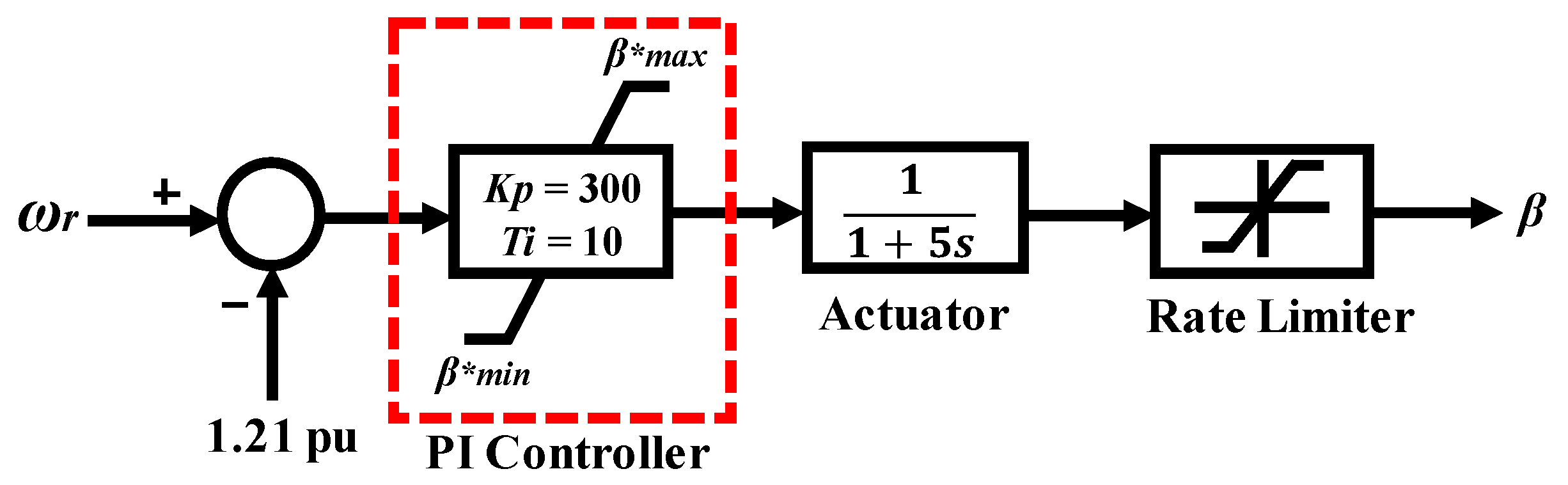Figure 4. Wind turbine characteristics for the doubly fed induction generator (DFIG) with maximum power point tracking (MPPT).
Figure 4. Wind turbine characteristics for the doubly fed induction generator (DFIG) with maximum power point tracking (MPPT).Figure 5. Configuration of the VSWT-DFIG system.
Figure 5. Configuration of the VSWT-DFIG system.Figure 6. Proposed rotor-side controller.
Figure 6. Proposed rotor-side controller.Figure 7. Grid-side controller.
Figure 7. Grid-side controller.Figure 8. Proposed fuzzy logic controller (FLC).
Figure 8. Proposed fuzzy logic controller (FLC).Figure 9. Internal structure of the FLC.
Figure 9. Internal structure of the FLC.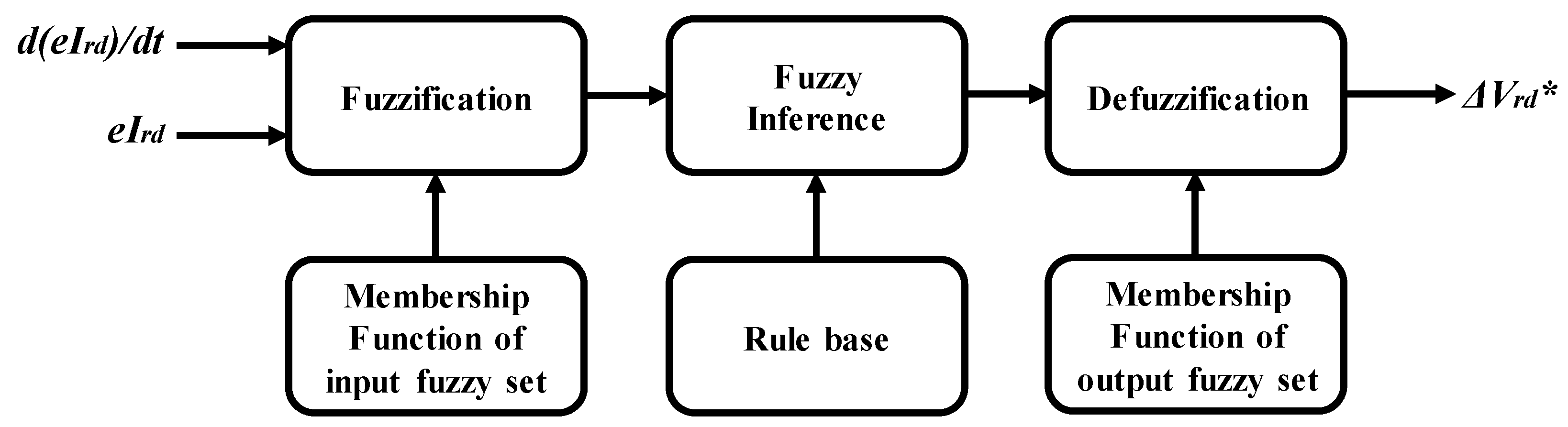Figure 10. Membership functions for the FLC: (a) Inputs (eIrd, d[eIrd]/dt)); (b) output (Vrd*).
Figure 10. Membership functions for the FLC: (a) Inputs (eIrd, d[eIrd]/dt)); (b) output (Vrd*).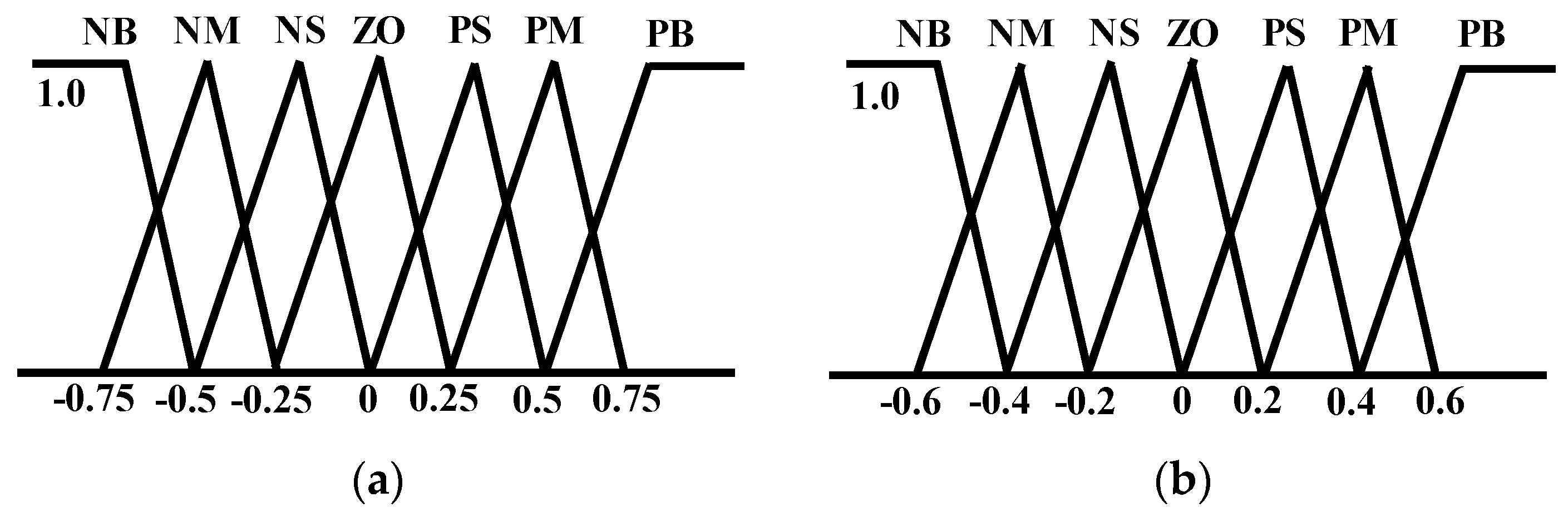Figure 11. Power system model.
Figure 11. Power system model.Figure 12. IEEE type AC4A excitation system model.
Figure 12. IEEE type AC4A excitation system model.Figure 13. Steam turbine governor model.
Figure 13. Steam turbine governor model.Figure 14. Hydro turbine governor model.
Figure 14. Hydro turbine governor model.Figure 15. Controller for automatic generation control (AGC).
Figure 15. Controller for automatic generation control (AGC).Figure 16. Low voltage ride through (LVRT) requirement for wind farm (WF).
Figure 16. Low voltage ride through (LVRT) requirement for wind farm (WF).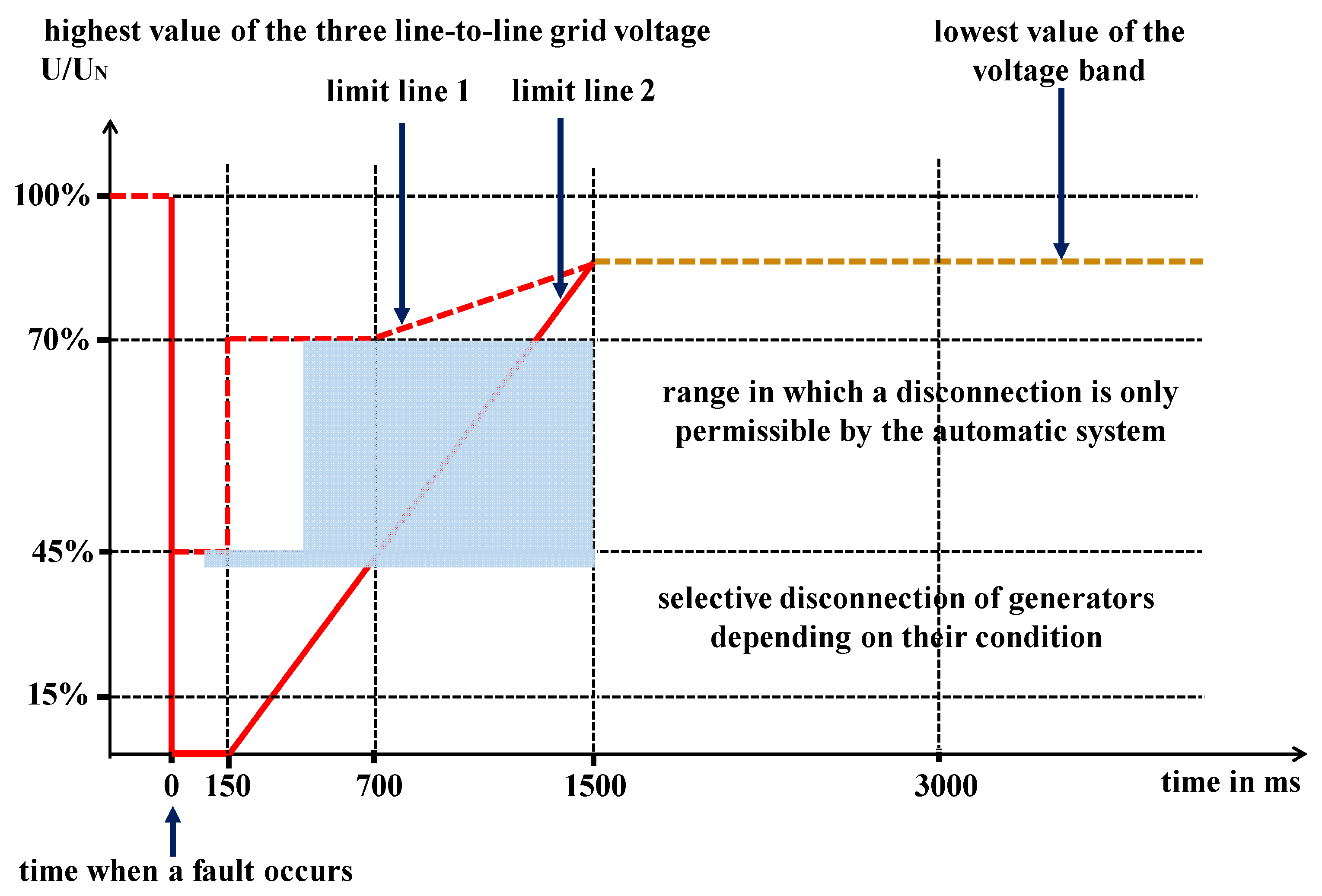Figure 17. Reactive power output of wind generators: (a) DFIG; (b) SCIG.
Figure 17. Reactive power output of wind generators: (a) DFIG; (b) SCIG.Figure 18. Individual response of the WF at bus 12: (a) Voltage at connection point; (b) total active and reactive power at connection point.
Figure 18. Individual response of the WF at bus 12: (a) Voltage at connection point; (b) total active and reactive power at connection point.Figure 19. Rotor speed response of wind generators: (a) DFIG; (b) SCIG.
Figure 19. Rotor speed response of wind generators: (a) DFIG; (b) SCIG.Figure 20. Active power output of wind generators: (a) DFIG; (b) SCIG.
Figure 20. Active power output of wind generators: (a) DFIG; (b) SCIG.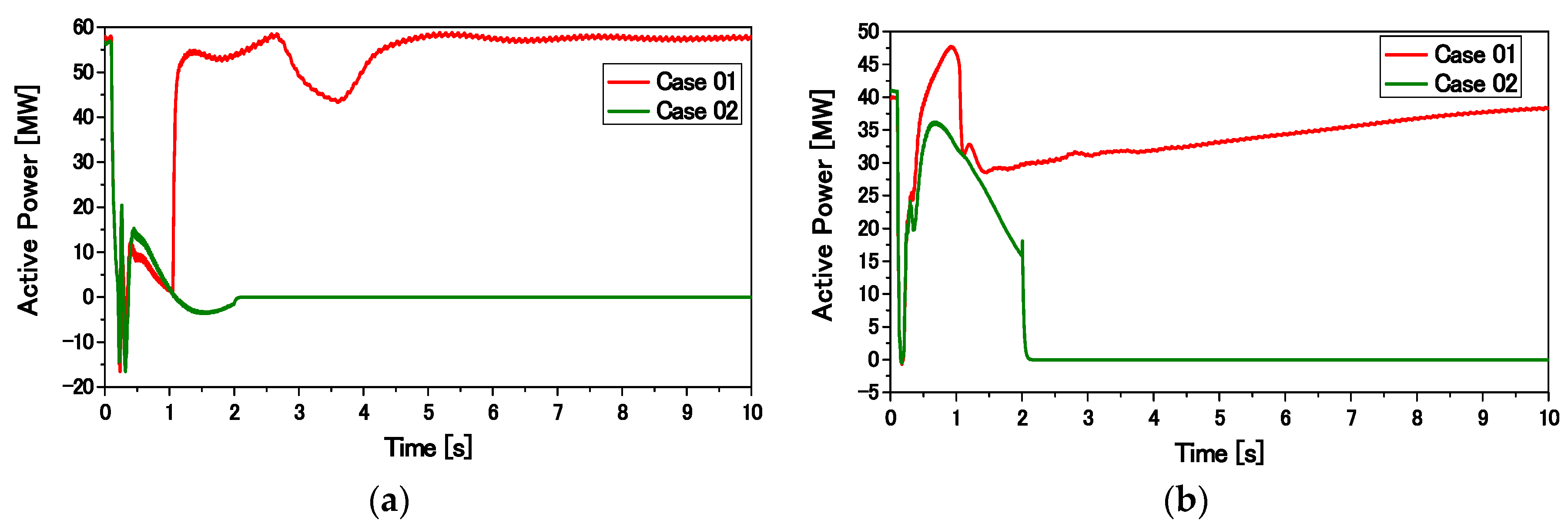Figure 21. Individual responses of the DFIG: (a) DC-link voltage; (b) reactive power output of GSC.
Figure 21. Individual responses of the DFIG: (a) DC-link voltage; (b) reactive power output of GSC.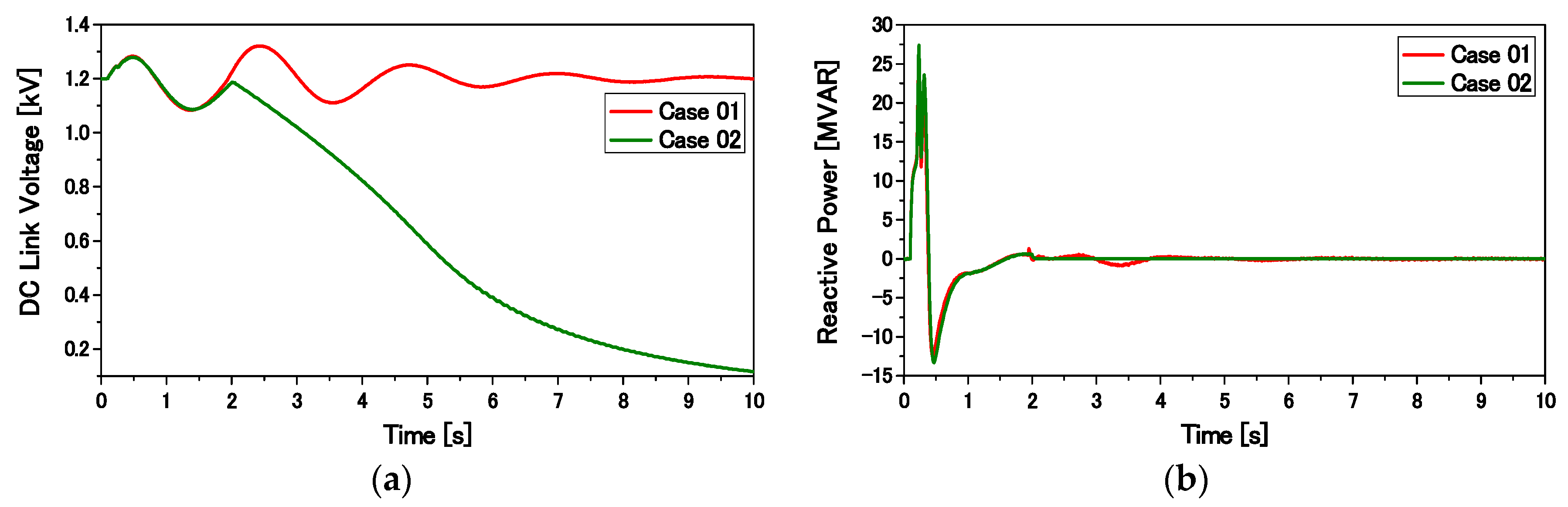Figure 22. Individual responses of conventional SGs: (a) active power; (b) rotor speed.
Figure 22. Individual responses of conventional SGs: (a) active power; (b) rotor speed.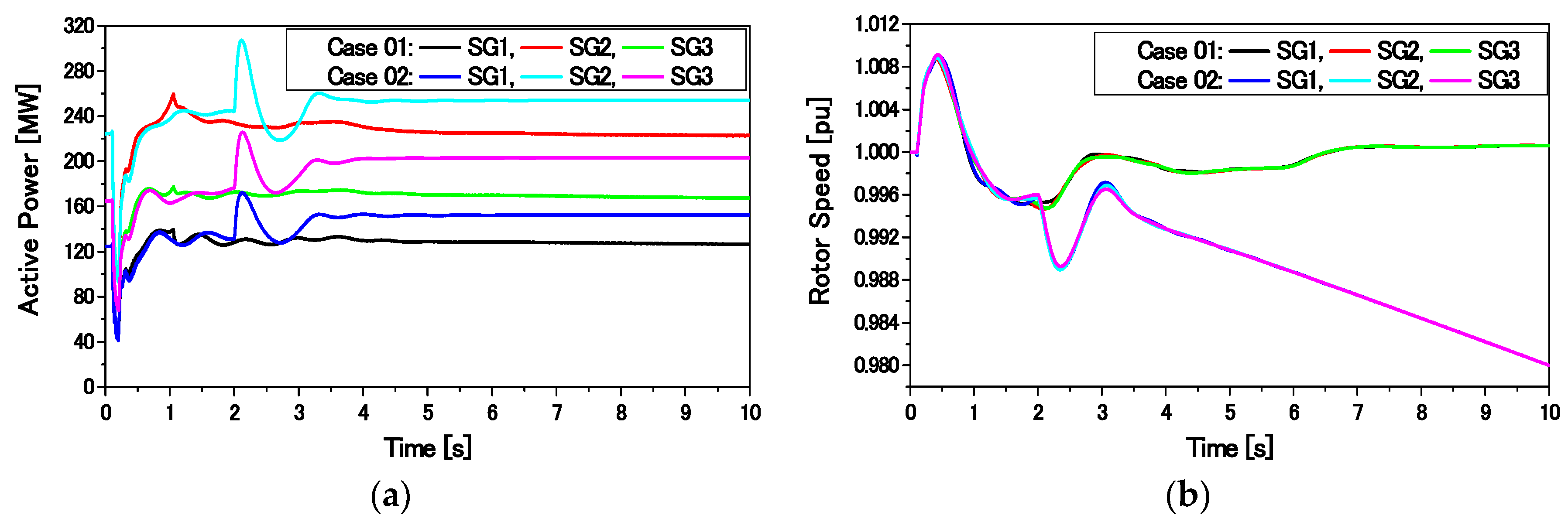Figure 23. Frequency responses of the power system.
Figure 23. Frequency responses of the power system.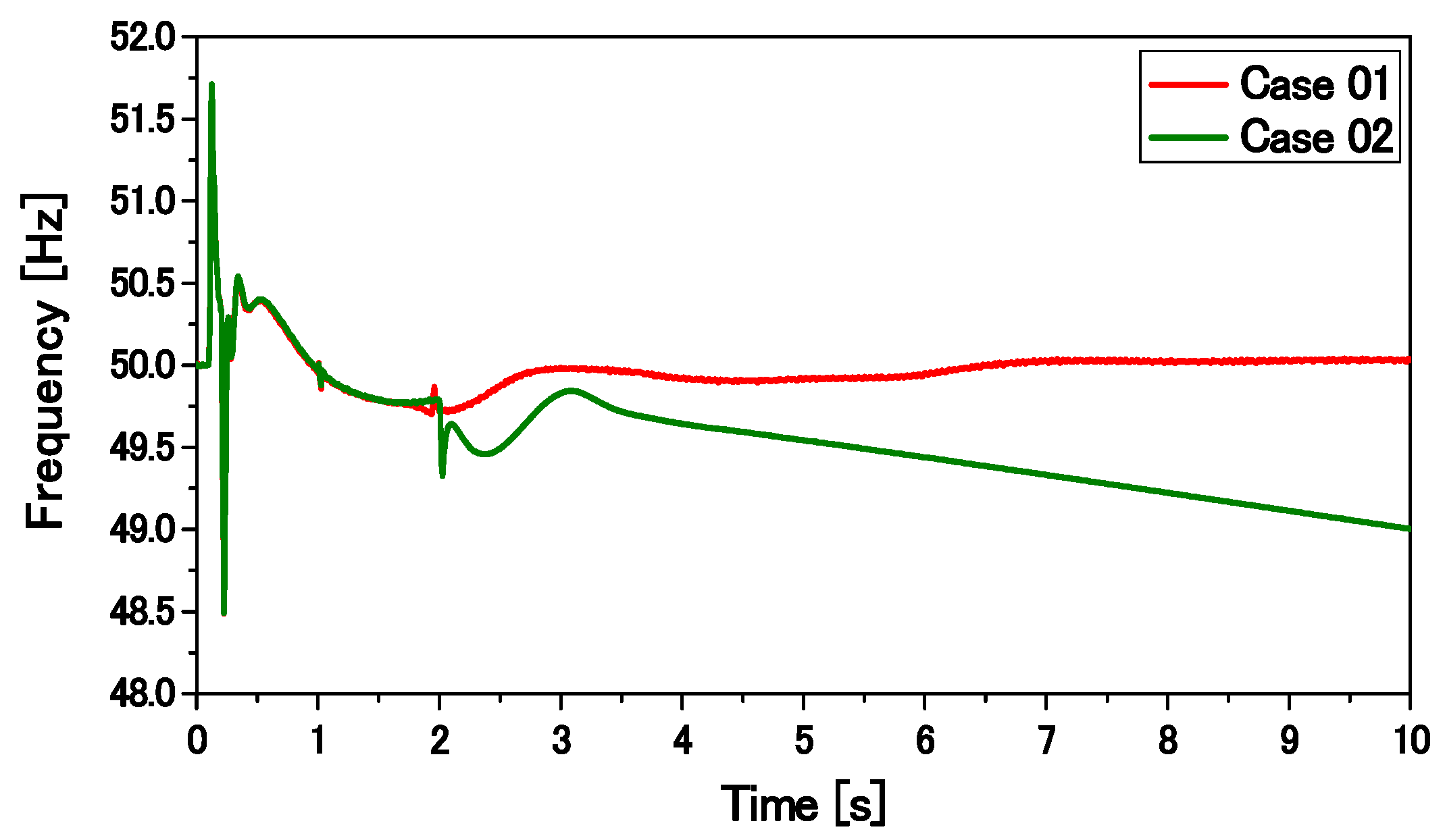Figure 24. Reactive power output of wind generators: (a) DFIG; (b) SCIG.
Figure 24. Reactive power output of wind generators: (a) DFIG; (b) SCIG.Figure 25. Individual response of the WF at bus 12: (a) Voltage at connection point; (b) total active and reactive power at connection point.
Figure 25. Individual response of the WF at bus 12: (a) Voltage at connection point; (b) total active and reactive power at connection point.Figure 26. Rotor speed response of wind generators: (a) DFIG; (b) SCIG.
Figure 26. Rotor speed response of wind generators: (a) DFIG; (b) SCIG.Figure 27. Active power output of wind generators: (a) DFIG; (b) SCIG.
Figure 27. Active power output of wind generators: (a) DFIG; (b) SCIG.Figure 28. Individual responses of the DFIG: (a) DC-link voltage; (b) reactive power output of GSC.
Figure 28. Individual responses of the DFIG: (a) DC-link voltage; (b) reactive power output of GSC.Figure 29. Individual responses of conventional SGs: (a) active power; (b) rotor speed.
Figure 29. Individual responses of conventional SGs: (a) active power; (b) rotor speed.Figure 30. Frequency responses of the power system.
Figure 30. Frequency responses of the power system.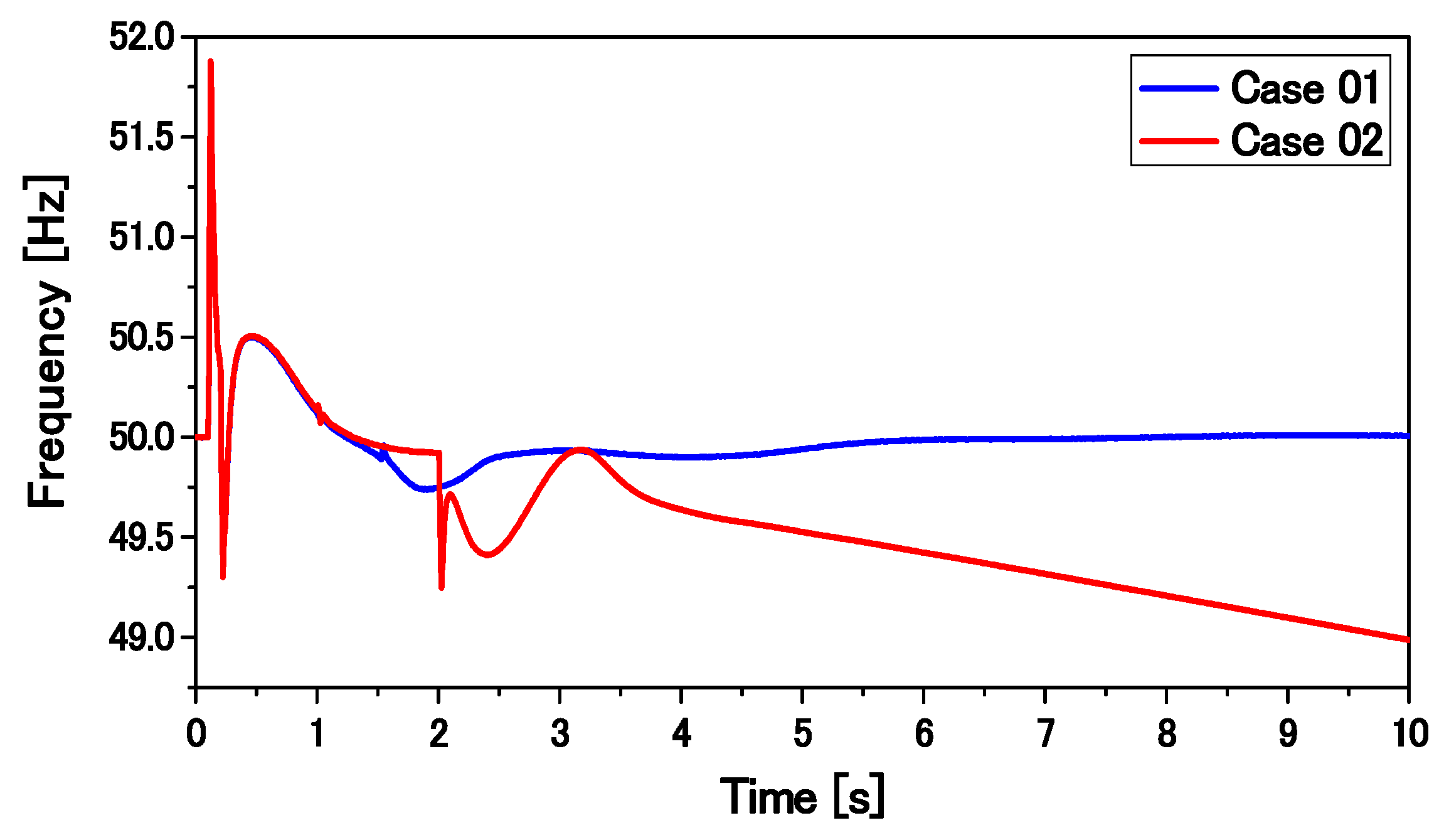Figure 31. Reactive power output of DFIG.
Figure 31. Reactive power output of DFIG.Figure 32. Wind speed data.
Figure 32. Wind speed data.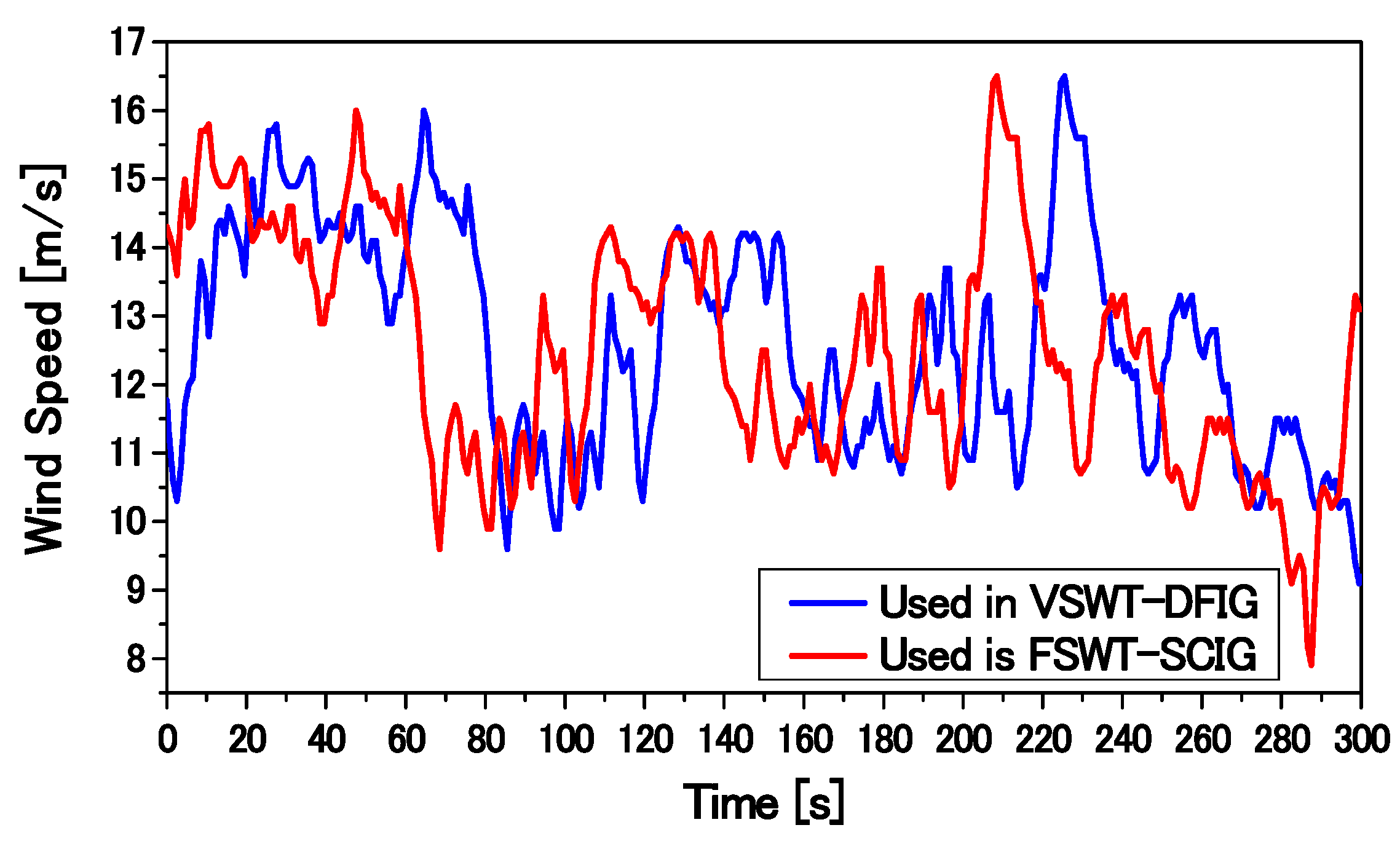Figure 33. Reactive power output of wind generators.
Figure 33. Reactive power output of wind generators.Figure 34. Voltage response at the connection point of wind generators.
Figure 34. Voltage response at the connection point of wind generators.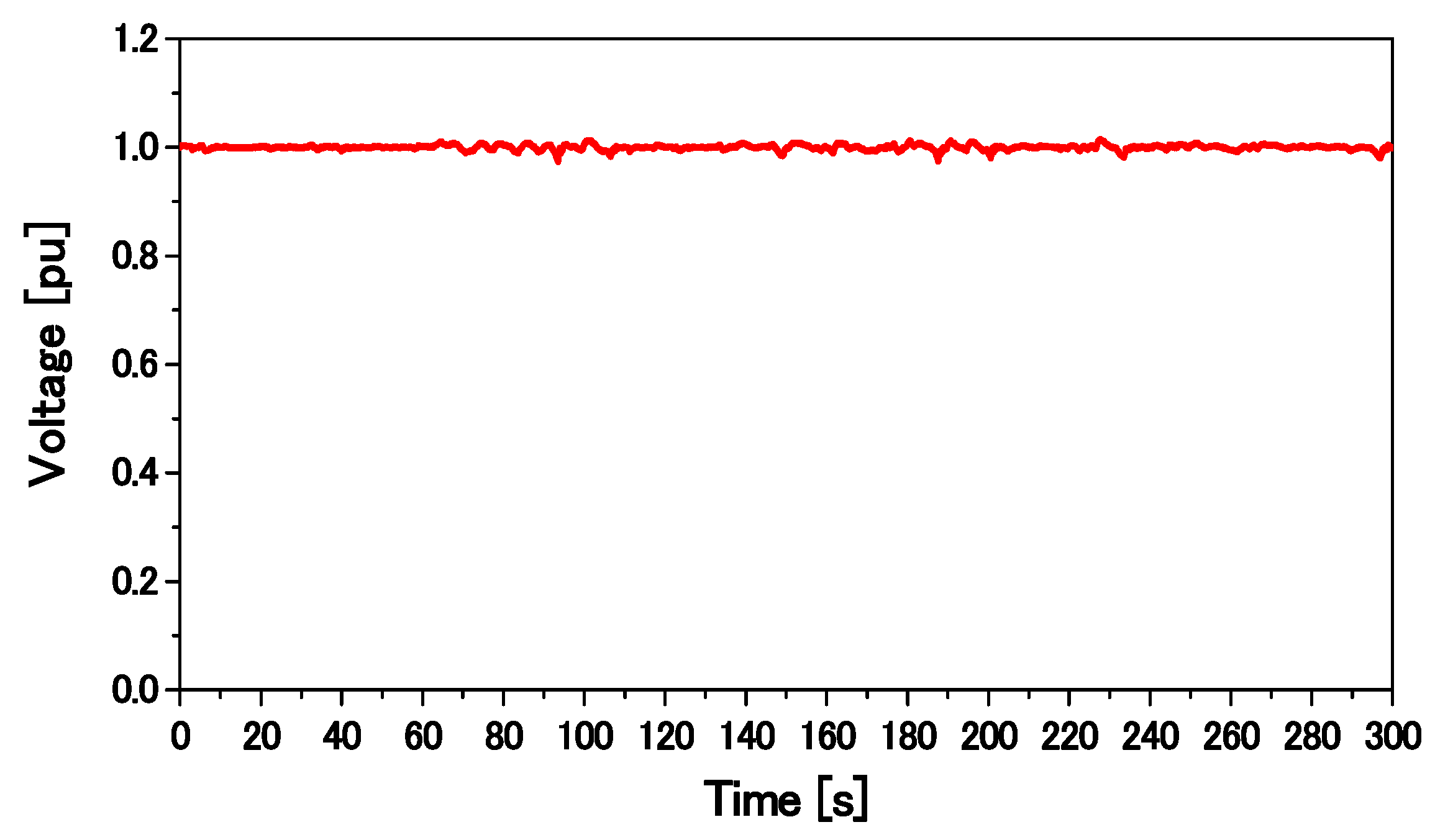Figure 35. Active power output of wind generators.
Figure 35. Active power output of wind generators.Figure 36. DC-link voltage response of DFIG.
Figure 36. DC-link voltage response of DFIG.Figure 37. Pitch angle of wind generators.
Figure 37. Pitch angle of wind generators.Figure 38. Total active and reactive power output at bus 12.
Figure 38. Total active and reactive power output at bus 12.Figure 39. Active power output of SGs.
Figure 39. Active power output of SGs.Figure 40. Power system frequency response.
Figure 40. Power system frequency response.Table 1. Fuzzy rules.
Table 1. Fuzzy rules.
Vrd*d(eIrd)/dt
PBPMPSZONSNMNB
eIrdPositive Big (PB)PBPBPMPMPSZOZO
Positive Medium (PM)PBPMPMPSZONSPS
Positive Small (PS)PMPMPSZONSNMPS
Zero (ZO)PMPSZONSNMNMPM
Negative Small (NS)PSZONSNMNMNBPM
Negative Medium (NM)ZONSNMNMNBNBPB
Negative Big (NB)NSNMNMNBNBNBPB
Table 2. Parameters of synchronous generators (SGs).
Table 2. Parameters of synchronous generators (SGs).
ParameterSG1 (Thermal)SG2 (Thermal)SG3 (Hydro)
Rated Power150 MVA250 MVA200 MVA
Voltage16.5 kV18 kV13.8 kV
Ra0.003 pu0.003 pu0.003 pu
Xl0.1 pu0.1 pu0.1 pu
Xd2.11 pu2.11 pu1.20 pu
Xq2.05 pu2.05 pu0.700 pu
X′d0.25 pu0.25 pu0.24 pu
X″d0.21 pu0.21 pu0.20 pu
X″q0.21 pu0.21 pu0.20 pu
T′do6.8 s7.4 s7.2 s
T″do0.033 s0.033 s0.031 s
T″qo0.030 s0.030 s0.030 s
H4.0 s4.0 s4.0 s
Table 3. Typical values of IEEE type AC4A excitation system.
Table 3. Typical values of IEEE type AC4A excitation system.
ParameterValue
KA200
TA0.04
TB12
TC1.0
Table 4. Typical values of turbine parameters.
Table 4. Typical values of turbine parameters.
Steam TurbineHydraulic Turbine
ParameterValueParameterValue
Rp0.05Rp0.05
TG0.2 sTG0.2 s
TCH0.3 sRT0.38 s
TRH7.0 sTR5.0 s
FHP0.3TW1.0 s
Table 5. Parameters of wind generators.
Table 5. Parameters of wind generators.
Doubly Fed Induction Generator (DFIG)Squirrel Cage Induction Generator (SCIG)
MVA27, 28, 58 and 59MVA41, 42, 72, 73
Rs0.007 puR10.01 pu
Rr0.005 puX10.1 pu
Lis0.171 puXm3.5 pu
Lrl0.156 puR210.035 pu
Lm2.9 puR220.014 pu
--X210.03 pu
--X220.089 pu
--H1.5 s
Table 6. Performances of the proposed and conventional rotor-side controllers of DFIG.
Table 6. Performances of the proposed and conventional rotor-side controllers of DFIG.
Controller for RSCDFIG (MW)SCIG (MW)Total Capacity of WF (MW)WF Condition (After the Fault)SGs Condition (After the Fault)
Proposed FLC2872100stablestable
Conventional PI controller5941100stablestable
Proposed FLC2773100unstableout of step
Conventional PI controller5842100unstableout of step